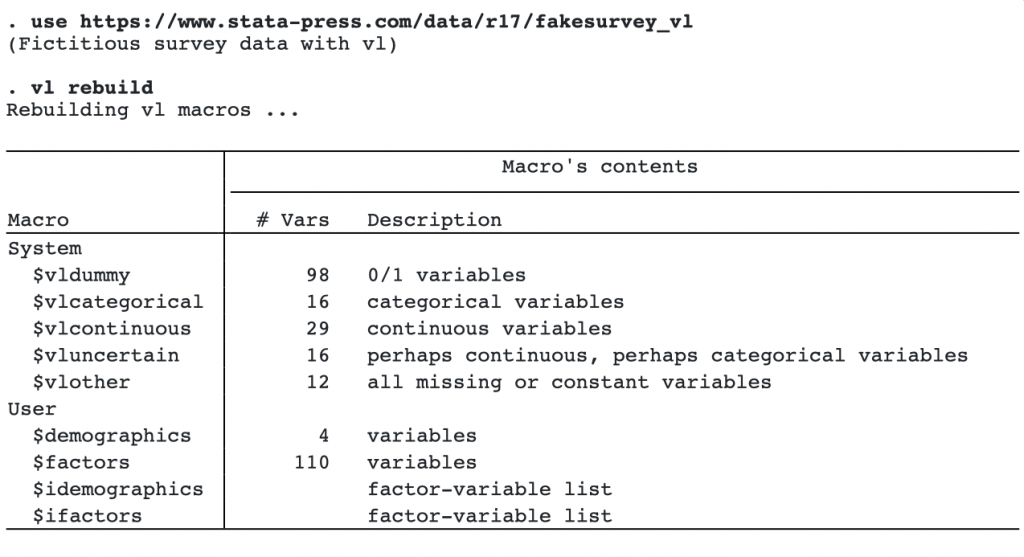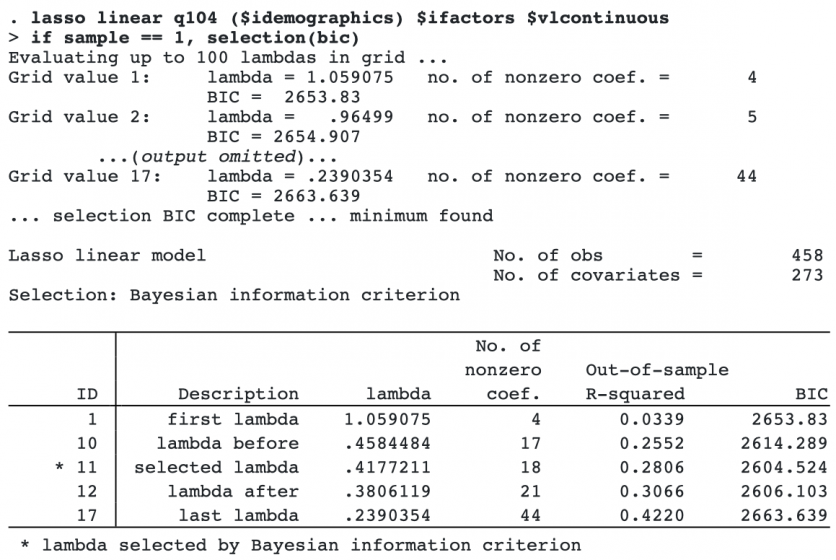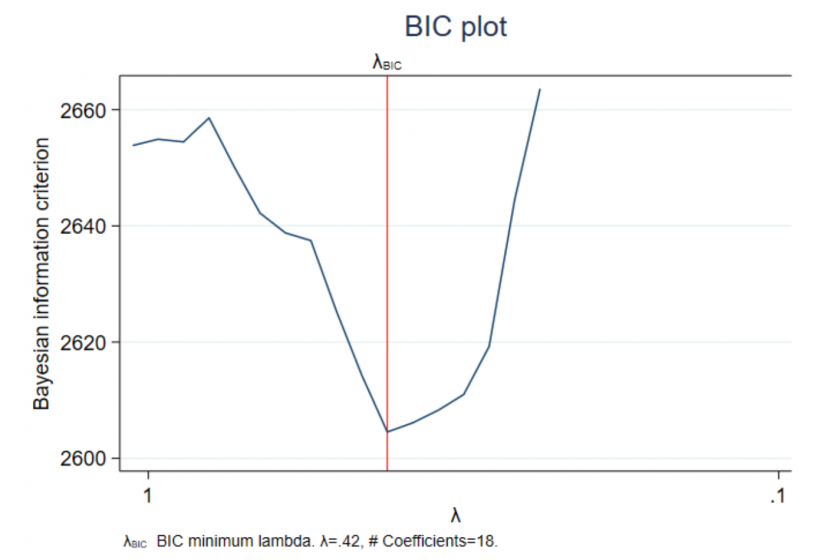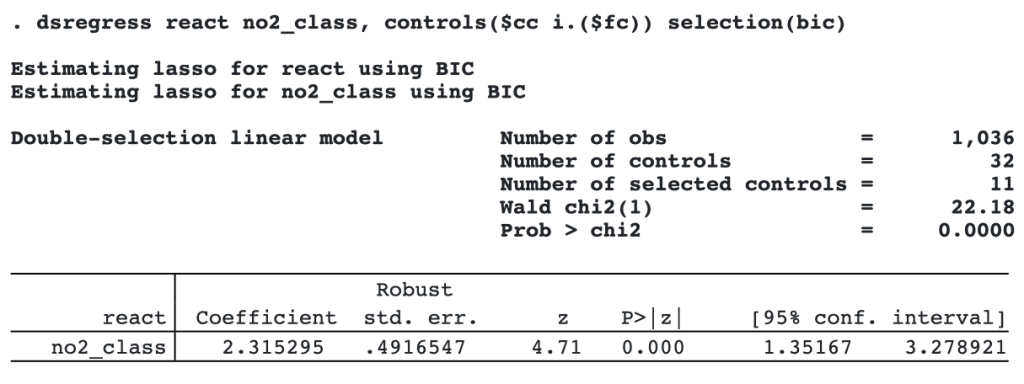## BIC for lasso penalty selection

Selection of the penalty parameter is fundamental to lasso analysis. Choose a small penalty parameter, and you risk including too many variables in your model. Choose a large one, and you might exclude important variables.

Now, we can use the Bayesian information criterion (BIC) to select the penalty parameters in lasso-related commands for both prediction and inference.

For prediction, we can choose the penalty parameters by minimizing BIC in lasso, elasticnet, and sqrtlasso. For inference, we can also choose penalty parameters by minimizing BIC in dsregress, dslogit, dspoisson, poregress, pologit, popoisson, poivregress, xporegress, xpologit, xpopoisson, xpoivregress, and telasso.

After lasso with BIC penalty parameter selection, we can plot the BIC function, which shows the values of the BIC criterion over the grid of penalty parameters. The plot also shows the minimum BIC, which is the value of the selected penalty parameter.

To choose the penalty parameters based on BIC, just specify option selection(bic).

For a linear model for y, with candidate covariates x1-x100, to use BIC for selection, we type

```. lasso linear y x1-x100, selection(bic)
```

To look at the fitted BIC function plot, we type

```. bicplot
```

Using double selection to estimate and test the effect of d1 on y, with control variables x1 to x100, is equally simple; we type

```. dsregress y d1, controls(x1-x100) selection(bic)
```

Again, we may use bicplot after.

## Using BIC in lasso for prediction

Datasets used with lasso typically have many variables. To get started, we use the variable management tool vl to save ourselves from typing many variable names manually.vl created a set of global macros, each one with a set of variables that we can use during estimation. vl makes life easier when you are dealing with large sets of covariates.

Next, we use splitsample to split the data into training data and testing data. The training data will be used to fit the lasso model, and the testing data will be used to evaluate the fitted model’s prediction performance.

```. set seed 12345671
. splitsample, generate(sample) nsplit(2)
. label define svalues 1 "Training" 2 "Testing"
. label values sample svalues
```

Now, we are ready to fit a lasso model by using BIC to select the penalty parameter. To do that, we need to specify the selection(bic) option.The penalty parameter selected by the minimum BIC criterion was 0.42.

We can look at the fitted BIC function plot by typing bicplot.

```. bicplot```

The BIC function decreases quickly before the minimum at λ=0.42.

## Using BIC in dsregress for inference

Suppose we are interested in knowing the effect of air pollution (no2_class) on childrens’ reaction time (react), controling for covariates. However, we are uncertain about which control variables to include in the model. We can use dsregress to consistently estimate the coefficient on no2_class while using lasso to select control variables.

We specify the selection(bic) option to use bic to select the penalty parameter in each lasso performed by dsregress. We include a set of 32 controls stored in the global macros cc and fc.We see that 11 of 32 controls are selected. Our point estimate for the effect of nitrogen dioxide on reaction time is 2.3, meaning that we expect reaction time to go up by 2.3 milliseconds for each microgram per cubic meter increase in nitrogen dioxide. This value is statistically different from 0.

dsregress actually ran two lassos, one for react and one for no2_class. We can plot the BIC function for both lassos by typing

`. bicplot, for(react)`

and

`. bicplot, for(no2_class)`